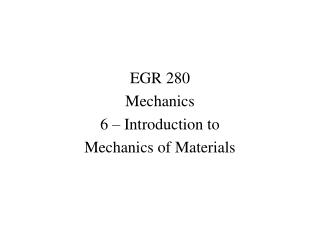DownloadDownload PresentationEGR 280 Mechanics 6 – Introduction to Mechanics of Materials

# EGR 280 Mechanics 6 – Introduction to Mechanics of Materials

Télécharger la présentation## EGR 280 Mechanics 6 – Introduction to Mechanics of Materials

- - - - - - - - - - - - - - - - - - - - - - - - - - - E N D - - - - - - - - - - - - - - - - - - - - - - - - - - -
##### Presentation Transcript

1. EGR 280 Mechanics 6 – Introduction to Mechanics of Materials

2. Introduction to Mechanics of Materials So far, we’ve looked at the forces applied to rigid bodies. • Of course, bodies deform when loads are applied to them • The deformation of bodies is a result of strain, which is the response of a material to stress • Stress is the intensity of loading that is experienced by a body, whether that body is rigid or not Loads applied to a body causes stress, which causes a strain in the material, which is measured as deformation of the body

3. Stress Let’s take a closer look at a truss member in tension: Cut through, or “section” the member into two parts: F F F F F P F P

4. The external forces F that come from the external loads are balanced by internal forces P. In the case shown above, the internal forces F are clearly equal to P, if the member is in static equilibrium. The internal forces must be resisted by the material of the truss member, else the member will fail. The forces P are distributed over areas within the body as stress, a measure of the force intensity σ = P/A σ = Normal stress P = Internal force A = Cross-sectional area F P F P P

5. Stress is the force per unit area, or the intensity of the force distributed over a given section. “Far” away from the points of loading, this axial stress is uniform across the cross-section (Saint-Venant’s theorem)

6. Near where the load is applied, the stress distribution is not uniform. As the section is moved away from the loading point, this type of stress, axial stress, becomes uniform over the cross-sectional area. Stress has units of force/area: lb/in2 = psi, N/m2 = Pa

7. Strain If we go back to our original truss member in tension, We know that as we pull on the member, it gets longer: Define the strain in the member as the deformation per unit length ε = δ/L F F F F L δ

8. Stress (σ=P/A) is a measure of the load intensity in the material, and strain (ε=δ/L) is a measure of the incremental deformation in the material. Plotting stress versus strain gives a curve that is characteristic of the material, and does not depend on the geometry of the specimen used Stress – strain diagram Obtained through a tensile-test, where a carefully-prepared specimen is placed into a testing machine which applies a gradually-increasing axial load. The deformation of the specimen is measured as it elongated and eventually breaks. True stress and strain As the tensile test specimen elongates, its diameter decreases. If we account for this change in diameter when we calculate the stress and strain, we can obtain true stress and strain in the specimen

9. Hooke’s Law: In the initial part of the stress-strain diagram, the stress is proportional to the strain, so that σ = E ε This is Hooke’s Law (Robert Hooke, English mathematician, 1635-1703). The proportionality coefficient E is the modulus of elasticity or Young’s modulus (English scientist Thomas Young, 1773-1829). If the axial stress σ does not exceed the proportional limit of the material, then σ = E ε P/A = E δ/L

10. So that the deformation of our truss member in tension is: δ = PL / AE F F L δ